Pattern Matching

The symbols Blank, BlankSequence, BlankNullSequence, Alternatives, Pattern, Optional, Repeated, RepeatedNull, Condition, PatternTest, Verbatim, HoldPattern, Default, Orderless, Flat, and OneIdentity are all related to pattern matching in Mathematica.  Each of these symbols are discussed in this notebook.

Some examples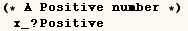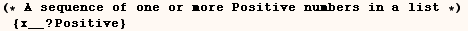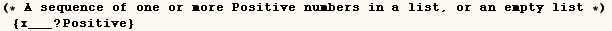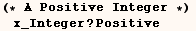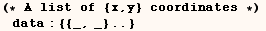A pattern must match the FullForm

Finding the right pattern needed to make a transformation on an algebraic  expression can be non-trivial. Consider the next cell where we would like to  make the transformation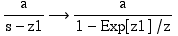on each term.
This is a simplified example of a real world problem I  encountered when I needed to change the transfer function of a continuous  filter to the transfer function (in the z-domain) of the corresponding  discrete filter. The most intuitive attempt to make this transformation is  shown in the next cell, and you can see it doesn't work.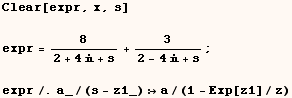The attempt above doesn't work because the pattern doesn't match the FullForm
of the expression we want to change.  The next cell indicates what the
FullForm is.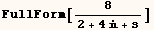The transformation in the next cell mathces the FullForm and does what we
want.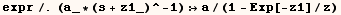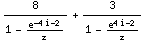For more examples in pattern matching see the sections on  MatchQ and HoldPattern.

Note:  The attributes Orderless, Flat, and OneIdentity have an effect on pattern matching.

The pattern matcher doesn't look inside "atoms"

Integers, rational numbers, and complex numbers are "atoms", and the
pattern matcher doesn't look at the individual parts of these things.  That
is why we get only only one Position from the next input.Keep in mind that the functions in the table below can be used to take apart
atoms.

Also be aware that the real and imaginary parts of a complex number can each
be Real, Rational, or Integer values.

 Head Function to take apart the atom Complex Re, Im Rational Numerator, Denominator Real RealDigits Integer IntegerDigits String Characters

The pattern (x_Symbol) may not be specific enough

In the next input a function f[x, n] is defined where x must be a symbol and
n must be an integer.Below we see that the above definition is used for f[π,3] and[s,3] when (s) evaluates to the symbol (t).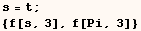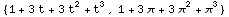However you might want to require that the first argument of (f) is a
variable and π is clearly not a variable.  The next definition does the
job.However, it gets a more complicated if you want to account for symbols  that were removed.  This was explained by Robby Villegas of Wolfram Research  at the 1999 Developer Converence.  See "Working With Unevaluated Expressions " posted at
http://library.wolfram.com/conferences/devconf99/#programming.

Below the symbol (t) is removed, so (s) evaluates to Removed["t"]. When f[s, 3] is evaluated the first argument Removed["t"] matches the  pattern (x_Symbol?(!NumericQ[#]&)). One would think Removed["t"] would be an expression with Head Removed  and argument "t", but it doesn't work that way.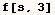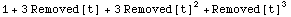In the next cell I give a new definition for (f) that requires that the first
argument is a non-numeric symbol that hasn't been Removed.You might find the function (UserSymbolQ) below useful.  It returns True when
it's argument is a Non-numeric symbol that hasn't been removed and is not in
the System Context.In the cell below the only "UserSymbol" is t4.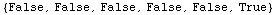Created by Mathematica  (May 17, 2004)